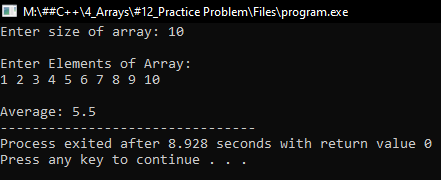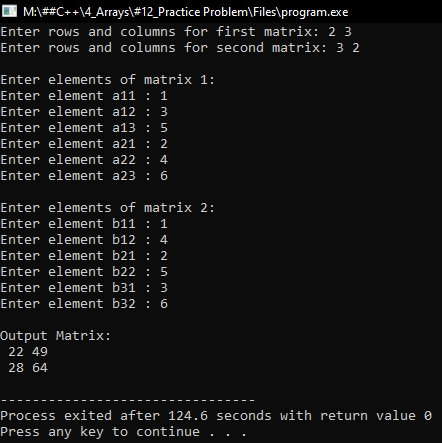# Array Practice Problems in C++

## Array Practice Problems in C++:

In this article, I am going to discuss Array Practice Problems in C++ with examples. Please read our previous articles, where we Multidimensional Array in C++ Language with examples.

##### Program to calculate the average of all the elements in an array using C++:
```#include <iostream>
using namespace std;
int main()
{
int n, m, sum = 0;
cout << "Enter size of array: ";
cin >> n;
int *A = new int[n];
cout << "\nEnter Elements of Array:\n";
for (int i = 0; i < n; i++)
{
cin >> A[i];
sum += A[i];
}
cout << "\nAverage: " << (float) sum / n;
}
```
###### Output:##### Program to Multiply 2 Matrices using C++:
```#include <iostream>
using namespace std;
int main()
{
int a, b, mult, r1, c1, r2, c2, i, j, k;
cout << "Enter rows and columns for first matrix: ";
cin >> r1 >> c1;
cout << "Enter rows and columns for second matrix: ";
cin >> r2 >> c2;

if (c1 != r2)
{
cout << "Cant be Multiplied";
return 0;
}

// Storing elements of first matrix.
cout << endl << "Enter elements of matrix 1:" << endl;
for (i = 0; i < r1; ++i)
for (j = 0; j < c1; ++j)
{
cout << "Enter element a" << i + 1 << j + 1 << " : ";
cin >> a[i][j];
}

// Storing elements of second matrix.
cout << endl << "Enter elements of matrix 2:" << endl;
for (i = 0; i < r2; ++i)
for (j = 0; j < c2; ++j)
{
cout << "Enter element b" << i + 1 << j + 1 << " : ";
cin >> b[i][j];
}

// Multiplying matrix a and b and storing in array mult.
for (i = 0; i < r1; ++i)
for (j = 0; j < c2; ++j)
{
mult[i][j] = 0;
for (k = 0; k < c1; ++k)
{
mult[i][j] += a[i][k] * b[k][j];
}
}

// Displaying the multiplication of two matrix.
cout << endl << "Output Matrix: " << endl;
for (i = 0; i < r1; ++i)
for (j = 0; j < c2; ++j)
{
cout << " " << mult[i][j];
if (j == c2 - 1)
cout << endl;
}

return 0;
}
```
###### Output: